Converting fractions to decimals isn’t difficult, but to do it, you need to know about decimal division. You also need to know how to deal with terminating and repeating decimals in your answer. Here are the steps to convert a fraction to a decimal:

1. Set up the fraction as a decimal division, dividing the numerator (top number) by the denominator (bottom number).

2. Attach enough trailing zeros to the numerator so that you can continue dividing until you find that the answer is either a terminating decimal or a repeating decimal.

## When the answer is a terminating decimal

Sometimes, when you divide the numerator of a fraction by the denominator, the division eventually works out evenly. The result is a terminating decimal. The following examples show terminating decimals.

Suppose you want to change the fraction 2/5 to a decimal. Here’s your first step: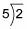One glance at this problem, and it looks like you’re doomed from the start because 5 doesn’t go into 2. But watch what happens when you add a few trailing zeros. Notice that you can also place another decimal point in the answer just above the first decimal point. This step is important: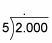Now you can divide because, although 5 doesn’t go into 2, 5 does go into 20 four times: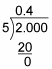You’re done! As it turns out, you only needed one trailing zero, so you can ignore the rest: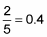Because the division worked out evenly, the answer is an example of a terminating decimal.

As another example, suppose you want to find out how to represent 7/16 as a decimal. First, you attach three trailing zeros: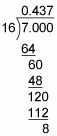In this case, three trailing zeros aren’t enough to get your answer, so you can attach a few more and continue: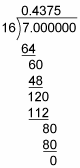At last, the division works out evenly, so again the answer is a terminating decimal. Therefore, 7/16 = 0.4375.

## When the answer is a repeating decimal

Sometimes when you try to convert a fraction to a decimal, the division never works out evenly. The result is a repeating decimal — that is, a decimal that cycles through the same number pattern forever.

You may recognize these pesky little critters from your calculator, when an apparently simple division problem produces a long string of numbers.

For example, to change 2/3 to a decimal, begin by dividing 2 by 3. Start out by adding three trailing zeros and see where it leads: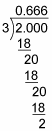At this point, you still haven’t found an exact answer. But you may notice that a repeating pattern has developed in the division. No matter how many trailing zeros you attach to the number 2, the same pattern will continue forever. This answer, 0.666 . . . , is an example of a repeating decimal. You can write 2/3 as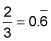The bar over the 6 means that in this decimal, the number 6 repeats forever. You can represent many simple fractions as repeating decimals. In fact, every fraction can be represented either as a repeating decimal or as a terminating decimal — that is, as an ordinary decimal that ends.

Now suppose you want to find the decimal representation of 5/11. Here’s how this problem plays out: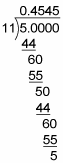This time, the pattern repeats every other number — 4, then 5, then 4 again, and then 5 again, forever. Attaching more trailing zeros to the original decimal will only string out this pattern indefinitely. So you can write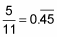This time, the bar is over both the 4 and the 5, telling you that these two numbers alternate forever.

Repeating decimals are an oddity, but they aren’t hard to work with. In fact, as soon as you can show that a decimal division is repeating, you’ve found your answer. Just remember to place the bar only over the numbers that keep on repeating.